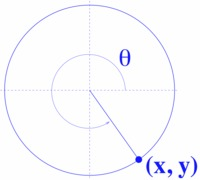# Finding Trig Values

Alignments to Content Standards: F-TF.C.8

Suppose that $\cos\theta = \frac{2}{5}$ and that $\theta$ is in the 4th quadrant. Find $\sin\theta$ and $\tan\theta$ exactly.

## IM Commentary

This is a very straight forward task that addresses the second part of F-TF.C.8 exactly. It could be used as an introductory example, practice or assessment.

The solution refers to a diagram involving the unit circle, which provides graphical intuition for the fact that the values of sine and tangent in the answer are negative. As such, this problem illustrates SMP 5 - Use Appropriate Tools Strategically and SMP 7 - Look for and Make Use of Structure.

## Solution

We have $\cos^2\theta + \sin^2\theta = 1$ and $\cos\theta= \frac{2}{5}.$ Therefore $\left(\frac{2}{5}\right)^2 + \sin^2\theta = 1$ and so $\sin^2\theta = 1 - \frac{4}{25} = \frac{21}{25}.$ Taking the square root we have $$\sin\theta = \pm \frac{\sqrt{21}}{5}.$$

Since $\theta$ is a 4th quadrant angle, the $y$-coordinate associated with the terminal side of $\theta$ is negative as shown in the diagram below.So $$\sin\theta \ =\ -\sqrt{21}/5.$$ Therefore, $$\tan\theta = \frac{\sin\theta}{\cos\theta} = \frac{-\sqrt{21}}{5} \cdot \frac{5}{2} \ = \ -\frac{\sqrt{21}}{2}.$$